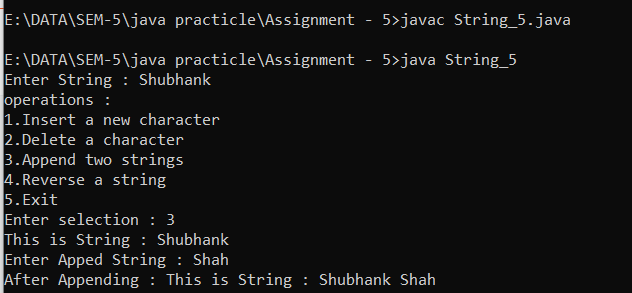• Parallel projection methods are used by engineers to create and working drawing of an object which preserves its true value.

• In the case of parallel projection the rays from an object coverage at infinity, unlike the perspective projection where the rays from an object converse at infinite distance.

• Parallel projection can be categorized according to the angle that projection of direction plan.

# Transformation of Parallel Projection

• Parallel projection (are also known as orthographic projection) is projections into one of the coordinate plans.

x = 0, y = 0 and z = 0

• The standard transformation parallel projection into the xy plane (i.e = z = 0 plans) is:

z = x' = x

y' = y

z' = 0

• In matrix form:

# Orthographic Parallel Equation

• When the projection lines are perpendicular to the view plane, we have an orthographic parallel equation.

• Orthographic projections are most often used to produce the front, side and top view of an object.

• Engineering and architecture drawings commonly used in orthographic projections.

• Because length and angle are accurately depicted and can be measure from the drawing.

• Transformation equations for an orthographic parallel projection are straight forward.

• Figure of orthographic parallel projection is given below:

# Oblique Parallel Projection

• An oblique projection is obtained by projecting points.

• Along parallel lines that are not perpendicular to the projection plane.

• Coordinates of oblique parallel projection can be obtained as:

• As shown in the above figure (x, y, z) is a point at which we are taking oblique projection (x, y), (y, p) on the view plane is an orthographic projection of (x, y, z).

xp = x + ∠cosθ

yp = y + ∠sinθ

∠ = z / tanx

so, tanx = z / ∠

Cohen Sutherland In Computer Graphics

3-D Rotation In Computer Graphics